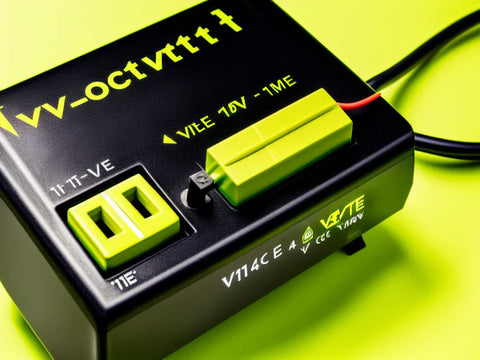kVA To Amps Calculator - ShopSolar.com

# kVA To Amps Calculator

The formulas and principles used in a kVA to amps calculator are similar to what you’d find in an mA to amps calculator since they both deal with amps.

Yes, the latter might be more straightforward, but when we have a standard variable that’s used in the calculations, it's easier to understand.

First things first, though, we should understand the variables we’re working with.

## kVA to Amps Calculator

 Enter phase #: Single phase Three phase Enter kilovolt-amps: kVA Select voltage type: Line to line voltage Line to neutral voltage Enter volts: V Result in amps: A

## What Is kVA?

When we talk about kVA (kilovolt-amps), we’re referring to the apparent power of a circuit or electrical system. In DC circuits, kVA and kW (kilowatts) are the same.However, real power, or kW, will differ from apparent power in AC circuits.

In this case, kW is the amount of actual power that does the work, but kVA is merely a fraction of the power that can do the job, while the rest is regarded as the current.

### Calculations

Calculating the kVA of a circuit is a simple formula and you only need to know the voltage and current. These are the same variables you need for a VA to amps calculator.

To work out kVA, multiply the voltage by the current and divide by 1,000.

For a more practical example with values, let’s say in a given circuit, we have a voltage of 420V, and the current flowing is 32A. Our calculation will look like this:

kVA = (V x A) / 1,000

kVA = (420 x 32) / 1,000

kVA = (13,440) / 1,000

13.44 kVA

When you’re using a house amp calculator to see where most of your power is being drawn from in your home, you may notice that the heavier appliances will have ratings in kVA.

But how do we convert this to amperes? More importantly, what are amps and where do we use them in our solar system?

## What Are Amps?

An amp is the amount of current flowing from one point to another.

This measurement can either be acquired by using an amp meter and connecting it to a point on the circuit, or it can be calculated.

1 A is equivalent to 1 coulomb per second and serves as the base unit of electric current.

Thanks to Ohm’s and Watt’s law, you can easily convert volts to amps or vice versa.

If you know two of the values in either of these laws, you can find the current by either multiplying or dividing the values together.

## Converting kVA to Amps

Where some calculators like the inverter amp draw calculator have a single formula to follow, converting kVA to amps is a bit different.

This conversion is possible through single- and three-phase loaded circuits, both of which have their own formula.

The formula for single-phase conversion states that multiplying the kVA by 1,000 and dividing that product by the voltage gives you the amperage.

So let’s put this formula to the test and plug in actual values.

If we have a kVA of 800 and a voltage of 230, and we’re trying to calculate the amps, slotting these values into our formula gives us the single-phase amps.

Eventually, we end up with 3,478.26 A.

The three-phase load amperage is a different formula that uses the same variables.

This formula states that the three-phase amperage is equivalent to the kVA multiplied by 1,000, dividing that product by 1.73, or the approximate square root of 3, and then multiplying it by the voltage.

Again, we should use numerical values to show how it works.

Let’s say we have a kVA of 800 again, but our voltage is now 420. We’re still trying to calculate the amperage.

We eventually end up with 1,101.01A as our three-phase amperage if we plugged in the correct values.

With these values, you can get a better understanding of appliances needed to be powered by your solar system, or better yet, sizing the battery of your DIY solar panel kit.

## Conclusion

The conversion process consists of two straightforward formulae that can be done manually or via an online KVA to Amps calculator.

Once you understand the two variables we’re working with when trying to convert one to another, it becomes much easier than simply rushing into it.

You can also make use of our solar load calculator to determine your needs.

You can find all of the solar & electrical calculators we have created in order to help you plan, size and install your own solar power system here.

## Join the Solar Lab!